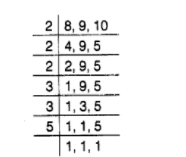# Find the smallest square number divisible

Question:

Find the smallest square number divisible by each one of the numbers 8, 9 and 10.

Solution:

The least number divisible by each of the numbers 8,9 and 10 is equal to the LCM of 8,9 and 10 .$\therefore \mathrm{LCM}$ of 8,9 and $10=2 \times 2 \times 2 \times 3 \times 3 \times 5=360$

Prime factors of $360=(2 \times 2) \times 2 \times(3 \times 3) \times 5$

Here, prime factors 2 and 5 are unpaired. Clearly, to make it a perfect square, it must be multiplied by $2 \times 5$, i.e. 10 .

Therefore, required number $=360 \times 10=3600$

Hence, the smallest square number divisible by each of the numbers 8,9 and 10 is 3600 .